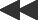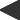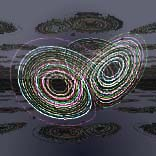basis: lorenz-fractal Lorenz 2 lobe attractor - orbit in 3 dimensions. in 2d the x and y components are projected to form the image. x(0) = y(0) = z(0) = 1; x(n + 1) = x(n) + (-a*x(n)*dt) + (a*y(n)*dt) y(n + 1) = y(n) + ( b*x(n)*dt) - (y(n)*dt) - (z(n)*x(n)*dt) z(n + 1) = z(n) + (-c*z(n)*dt) + (x(n)*y(n)*dt) parameters are dt, a, b, and c.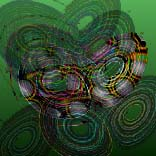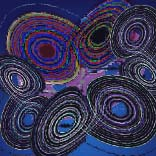The "Lorenz Attractor" is a "simple" set of three deterministic equations developed by Edward Lorenz while studying the non- repeatability of weather patterns. The weather forecaster's basic problem is that even very tiny changes in initial patterns ("the beating of a butterfly's wings" - the official term is "sensitive dependence on initial conditions") eventually reduces the best weather forecast to rubble.